## Example Questions

2 Next →

### Example Question #11 : How To Find Decimal Fractions

Convert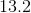to an improper fraction.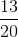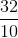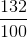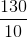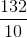Explanation:

First convert this decimal number to a mixed number fraction, then covert the mixed number to an improper fraction. The mixed number fraction must have a whole number ofand a fraction that representstenths. Then, convert the mixed number to an improper fraction by multiplying the denominator by the whole number, and then add that product to the numerator.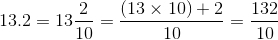### Example Question #12 : How To Find Decimal Fractions

Convert the mixed fraction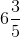to a decimal number.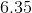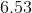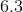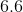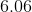Explanation:

Since the starting number is a mixed number fraction, the decimal number must also have a value in the ones place. Since the denominator in the fraction has a value that is a factor of, both the numerator and denominator can be multiplied by a factor ofto formtenths. (Note, the first decimal place value is the tenths place).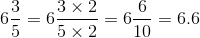### Example Question #51 : Decimals

A retailer can only order wristbands in bulk cases. Each case has 300 wristbands and costs $1172. Quantity A: The number of wristbands that can be bought for$10547.

Quantity B: The number of wristbands that can be bought for \$10560.

The relationship cannot be determined.

Quantity B is greater.

The two quantities are equal.

Quantity A is greater.

Quantity B is greater.

Explanation:

The key to this problem is to realize that the store cannot buy partial crates of wristbands--it's all or nothing. Calculate how many crates can be bought with each sum of money by dividing the sum by the price of a crate.

Quantity A: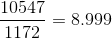Although oh so close, only eight crates can be bought with this sum of money. Don't round up!

Quantity B: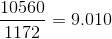There's just enough to buy nine crates.

Quantity B is greater.

2 Next →

### All GRE Math Resources